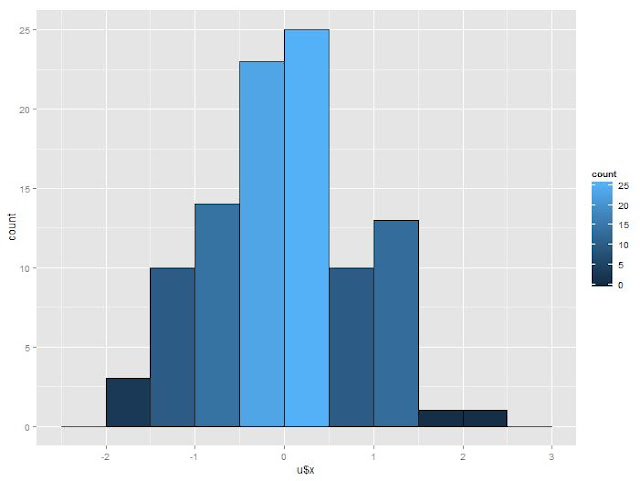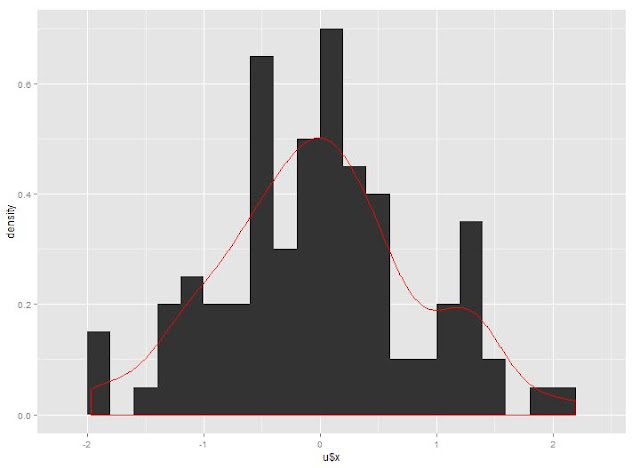## Saturday, December 8, 2012

### R: Replicate plot with ggplot2 (part 2) histogram, emprtical curve, normal density curve

The first one is to replicate the scatter plot here. This part is to replicat the plot of histogram.

The original UCLA ATS like is: http://www.ats.ucla.edu/stat/r/gbe/histogram.htm.

In ggplot2, geom_histogram is used to draw histogram.

The data is:

The first one is histogram with black fill:

The output graph is:ggplot2 has more choice that you can fill in the color by the counts in each bin. likeNext is to change the binwidth. In ggplot2 this can be done by binwidth or by breaks. Here is shown by binwidth:Next is to change from frequency to density(percentage in fact). This can be done by assigning y to be ..density.. in ggplot2:Then is to add normal density and empirical curve in the plot.

First is the empirical curve:Next is the Normal density with mean and std are from the generated data:The last one is to add the counts on top of each bin. I did not figure out how to do it. It should be easy by adding text. But unitl now my process has not reached there.